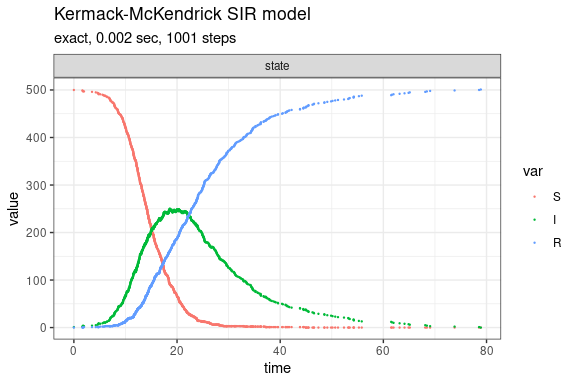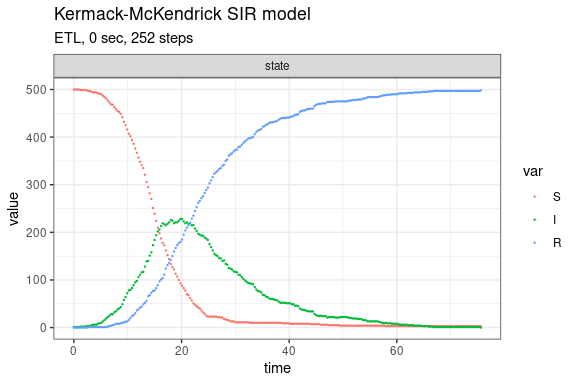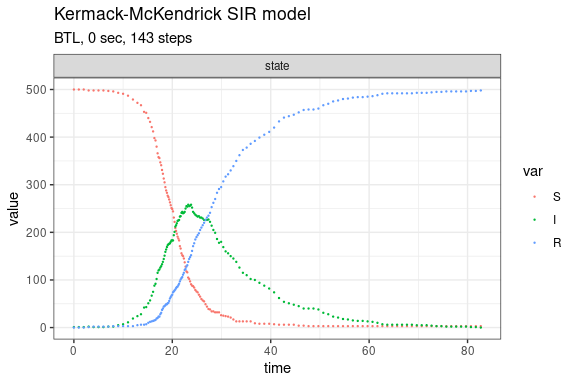# Kermack-McKendrick SIR model (Brown & Rothery, 1993)

The Kermack-McKendrick SIR model is defined as

dS/dt = -beta*N*S
dI/dt = beta*N*S - gamma*I
dR/dt = gamma*I

Note that simulations of this model can generate in all zero propensity, if the first reaction is a recovery of the single ‘Infected’ individual.

Define parameters

library(GillespieSSA2)
sim_name <- "Kermack-McKendrick SIR model"
params <- c(beta = .001, gamma = .1)
final_time <- 100
initial_state <- c(S = 500, I = 1, R = 0)

Define reactions

reactions <- list(
reaction("beta * S * I", c(S = -1, I = +1), name = "transmission"),
reaction("gamma * I", c(I = -1, R = +1), name = "recovery")
)

Run simulations with the Exact method

set.seed(1)
out <- ssa(
initial_state = initial_state,
reactions = reactions,
params = params,
final_time = final_time,
method = ssa_exact(),
sim_name = sim_name
)
plot_ssa(out)Run simulations with the Explict tau-leap method

set.seed(1)
out <- ssa(
initial_state = initial_state,
reactions = reactions,
params = params,
final_time = final_time,
method = ssa_etl(),
sim_name = sim_name
)
plot_ssa(out)Run simulations with the Binomial tau-leap method

set.seed(2)
out <- ssa(
initial_state = initial_state,
reactions = reactions,
params = params,
final_time = final_time,
method = ssa_btl(),
sim_name = sim_name
)
plot_ssa(out)